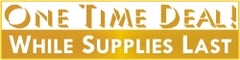# Casio Natural - V.P.A.M. Scientific Calculator - 2nd Edition (FX-300ES Plus) Select Color

Casio Computer Co. Ltd.

Regular price \$19.99 \$10.00 Sale

###Product Features:

• Pack of 1 Scientific Calculator with Slide-on Hard Case
• Great for General math, pre-algebra, algebra I & II, geometry, trigonometry, statistics, science, biology
• Binary, Octal, Decimal & Hexadecimal calculation
• Permitted on AP, SAT, SAT Subject, ACT & PSAT/NMSQT
• Also approved for AP Physics, AP Chemistry, AP Calculus
• Converts between Standard form and a Decimal
• Review/edit previous entries
• Random Number Generator
• 2-function Table of values [f(x), g(x)]
• Statistics and Linear Regression
• Integer Division with a Remainder
• Greatest Common Divisor, Least Common Multiple
• Memory & statistic calculation and more
• Solar power with battery back-up
• Total Size: approx. 6.375 in. x 3.125 in. x  .625 in.
• Select Color